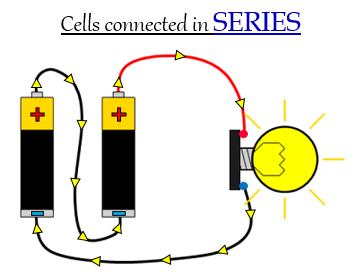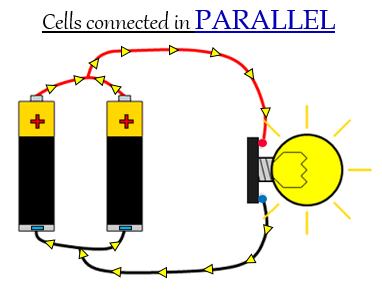# Describe how two cells can be connected in series and in parallel with the help of a suitable diagram.

Series Connection: It is the connectivity of multiple cells in which the positive terminal of one battery is connected to the negative terminal of the next battery (and this repeats if there are more than two batteries).

In this connection, the electrical circuit, components are arranged in a line, and the same current flows through all the components in the circuit. The current in this connection has only one path.

When the resistors are placed in a series circuit, the voltage across each resistor is different, even though the current flow is the same through them all. If one component breaks down in this connection, the whole circuit will burn out.Parallel Connection: It is the connectivity of multiple cells in which all of the positive battery terminals are connected together, and all of the negative battery terminals are connected together.

In this connection, the electrical circuit, components are arranged parallel to each other, and the electric current has multiple paths to flow through. This connection has a constant voltage across all ends.

When the resistors are placed in a parallel circuit, the voltage of each resistor is the same, and even polarity is the same. Even if one component breaks down in this connection, then the other components will function properly as each has its own independent circuit.Updated on: 10-Oct-2022

53 Views